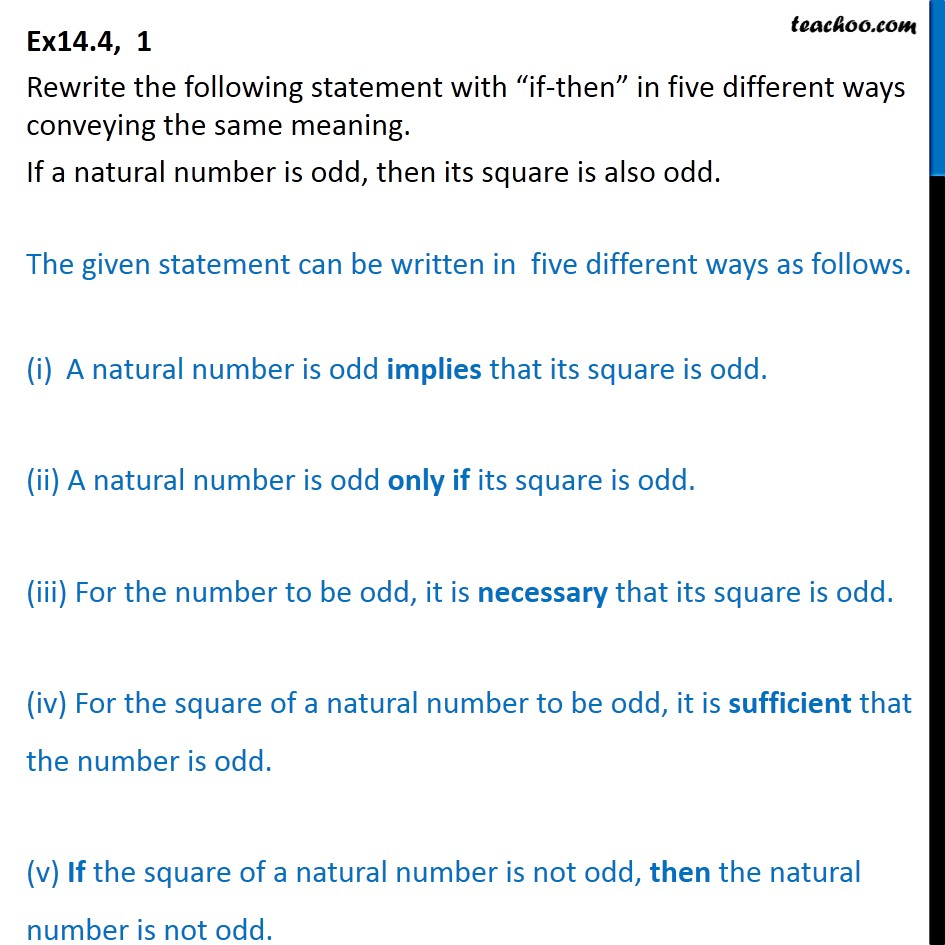If then Statements

Mathematical Reasoning
Serial order wiseLearn in your speed, with individual attention - Teachoo Maths 1-on-1 Class

### Transcript

Question 1 Rewrite the following statement with “if-then” in five different ways conveying the same meaning. If a natural number is odd, then its square is also odd. The given statement can be written in five different ways as follows. A natural number is odd implies that its square is odd. (ii) A natural number is odd only if its square is odd. (iii) For the number to be odd, it is necessary that its square is odd. (iv) For the square of a natural number to be odd, it is sufficient that the number is odd. (v) If the square of a natural number is not odd, then the natural number is not odd.